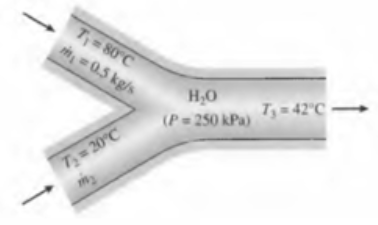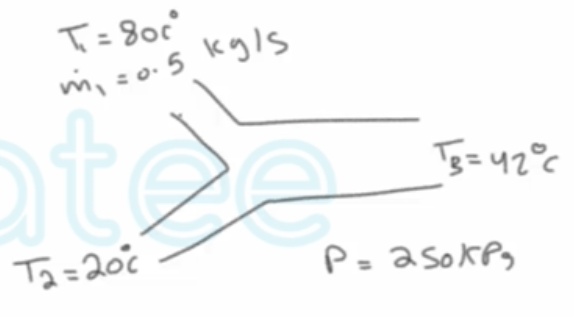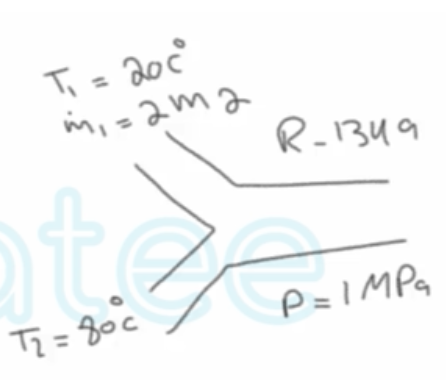Need Help?

Subscribe to Thermodynamics

###### \${selected_topic_name}
• Notes

$\begin{array}{l}{\text { A hot-water stream at } 80^{\circ} \mathrm{C} \text { enters a mixing chamber with a mass flow rate of } 0.5 \mathrm{kg} / \mathrm{s}} \\ {\text { where it is mixed with a stream of cold water at } 20^{\circ} \mathrm{C} \text { . If it is desired that the mixture leave }} \\ {\text { the chamber at } 42^{\circ} \mathrm{C} \text { , determine the mass How rate of the cold-water stream. }} \\ {\text { Assume all the streams are at a pressure of } 250 \mathrm{kPa} \text { . }}\end{array}$$\text { Mixing chamber }$

$P=\text {constant }$

$\dot{W}=Z e r o$

$\dot{m}_{1}+\dot{m}_{2}=\dot{m}_{3}$$T_{sat} \ @ \ 250 kPa=127.41 {c^{\circ}}$

$T<T_{\text {sat }}$

$h_{1}=h _f \ @\ 80 c^{\circ}=335.02 kJ/ kg$

$h_{2}=h_{f} @\ {20} {c^{\circ}}=83.915 kJ/kg$

$h_{3}=h_{f} \ @ \ {42} c^{\circ}=175.9 kJ/kg$

$\dot{m}_{\text {in }}-\dot{m}_{\text {out }}=\Delta \dot{m}_{\text {system }}=0\longrightarrow\dot m_1+\dot m_2=\dot m_3$

$\dot{E}_{\text {in }}=\dot{E} _\text { out } \longrightarrow \dot m, h_1+\dot m_2 h_2=\dot m_3 h_3$

$\dot m_{1} h_{1}+\dot m_{2} h_{2}=\left(\dot m_{1}+\dot{m}_{2}\right) h_{3}$

$\rightarrow \dot m_{2}=\frac{h_{1}-h_{3}}{h_{3}-h_{2}}\left(\dot {m}_{1}\right)$$=\frac{335 \cdot 02-175 \cdot 9}{175 \cdot 9-83.915}(0.5)=0.865 kg/s$

$\begin{array}{l}{\text { A stream of refrigerant- } 134 a \text { at } 1 \text { MPa and } 20^{\circ} \mathrm{C} \text { is mixed with another stream at } 1 \mathrm{MPa} \text { and } 80^{\circ} \mathrm{C} \text { . }} \\ {\text { If the mass flow rate of the cold stream is twice that of the hot one, }} \\ {\text { determine the temperature and the quality of the exit stream. }}\end{array}$$A-11,12,13$

$h_{1} \ @ \ h_{f20c^{\circ}}=79.32 kJ/kg$

$h_{2} \ @ \ h_{f/MPa 80c^{\circ}}=314.25 kJ/kg$

$\dot{m}_{1}+\dot{m}_{2}=\dot{m}_{3}$

$2 \dot m_{2}+\dot{m}_{2}=\dot{m}_{3} \longrightarrow 3\dot m_2=\dot m_3$

$\dot{E}_{i n}=\dot{E}_{out} \rightarrow$$\dot m_{1} h_{1}+\dot m_{2} h_{2}=\dot m_{3} h_{3}$

$\longrightarrow 2 \dot m _2 h_{1}+\dot m_2 h_2=3 \dot m_2 h_3$

$\rightarrow h_{3}=\frac{2 h_{1}+h_{2}}{3}=$$\frac{2(79.32)+314.25}{3}$

$∴ h_{3}=157.63 \mathrm{kJ} / \mathrm{kg}$

$@ \ 1 \mathrm{MPa} \rightarrow \mathrm{h_f}<\mathrm{h}_{3}<\mathrm{h_g}\quad ∴ S.M$

$T_{3}=T_{sulf} @ \ 1MPa=39.37 c^{\circ}$

$X_{3}=\frac{h_{3}-h_ f}{h_{fg}}=$$\frac{157.63-107.32}{270.99-107.32}=0.307$